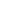Division Worksheets

• Detail View
• List View

Long Division Worksheets (with and without Remainders)

Worksheets of long division (with and without Remainders)

Mixed Division Worksheets (with and without Remainders)

Worksheets of mixed division (with and without Remainders)

Single Digit Quotient Simple Division Worksheets (with Remainders)

Worksheets of single digit quotient division (with Remainders)

Single Digit Quotient Simple Division Worksheets (No Remainders)

Worksheets of single digit quotient division (with no Remainders)

Visual Division Worksheets (With Remainders)

Worksheets of visual division (with Remainders)

Visual Division Worksheets (No Remainders)

Worksheets of visual division (no Remainders)